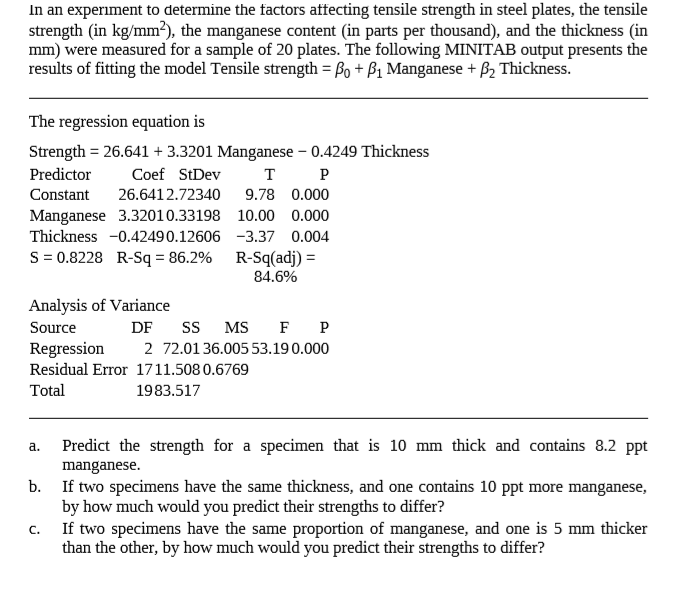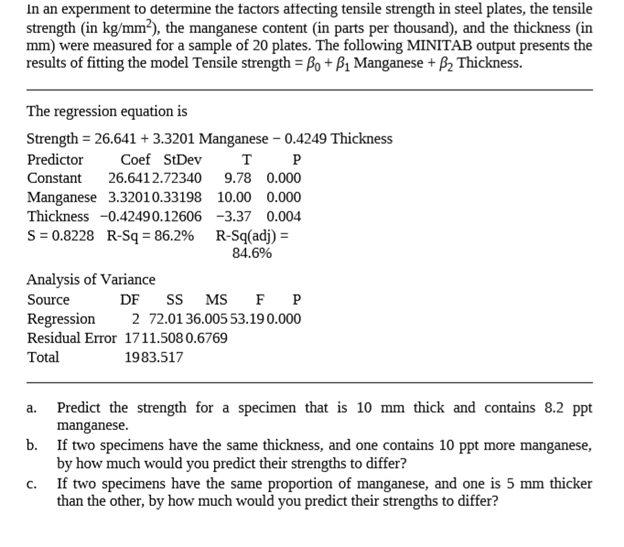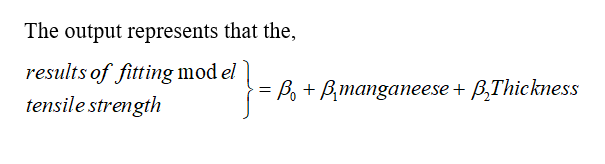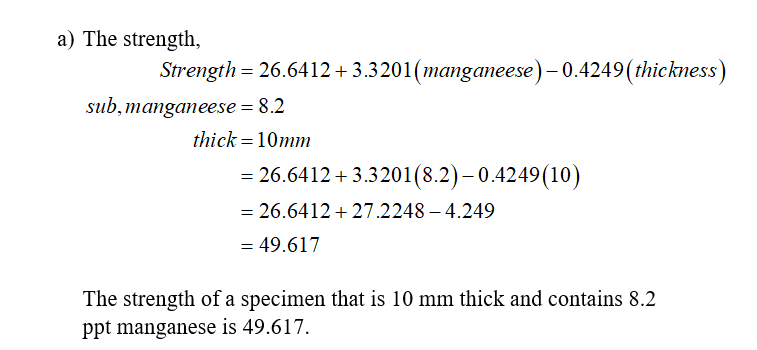# In an experiment to determine the factors affecting tensile strength in steel plates, the tensilestrength (in kg/mm?), the manganese content (in parts per thousand), and the thickness (inmm) were measured for a sample of 20 plates. The following MINITAB output presents theresults of fitting the model Tensile strength = Bo + B1 Manganese + B2 Thickness.The regression equation isStrength = 26.641 + 3.3201 Manganese – 0.4249 ThicknessPredictorCoef StDevт РConstant26.6412.723409.78 0.000Manganese 3.32010.3319810.00 0.000Thickness -0.42490.12606 -3.37 0.004S = 0.8228 R-Sq = 86.2% R-Sq(adj) =84.6%Analysis of VarianceSS MS2 72.0136.005 53.190.000SourceDFRegressionResidual Error 1711.508 0.6769Total1983.517Predict the strength for a specimen that is 10 mm thick and contains 8.2 ppta.manganese.b. If two specimens have the same thickness, and one contains 10 ppt more manganese,by how much would you predict their strengths to differ?If two specimens have the same proportion of manganese, and one is 5 mm thickerthan the other, by how much would you predict their strengths to differ?C.

Question
1 viewshelp_outlineImage TranscriptioncloseIn an experiment to determine the factors affecting tensile strength in steel plates, the tensile strength (in kg/mm?), the manganese content (in parts per thousand), and the thickness (in mm) were measured for a sample of 20 plates. The following MINITAB output presents the results of fitting the model Tensile strength = Bo + B1 Manganese + B2 Thickness. The regression equation is Strength = 26.641 + 3.3201 Manganese – 0.4249 Thickness Predictor Coef StDev т Р Constant 26.6412.72340 9.78 0.000 Manganese 3.32010.33198 10.00 0.000 Thickness -0.42490.12606 -3.37 0.004 S = 0.8228 R-Sq = 86.2% R-Sq(adj) = 84.6% Analysis of Variance SS MS 2 72.0136.005 53.190.000 Source DF Regression Residual Error 1711.508 0.6769 Total 1983.517 Predict the strength for a specimen that is 10 mm thick and contains 8.2 ppt a. manganese. b. If two specimens have the same thickness, and one contains 10 ppt more manganese, by how much would you predict their strengths to differ? If two specimens have the same proportion of manganese, and one is 5 mm thicker than the other, by how much would you predict their strengths to differ? C. fullscreen
check_circle

Step 1

Given that,Step 2Step 3...

### Want to see the full answer?

See Solution

#### Want to see this answer and more?

Solutions are written by subject experts who are available 24/7. Questions are typically answered within 1 hour.*

See Solution
*Response times may vary by subject and question.
Tagged in